# FREQUENCY TO VOLTAGE CONVERTER CIRCUITS

Frequency to voltage converter is an electronic device which converts the sinusoidal input frequency into a proportional current or output voltage.The basic circuit includes operational amplifiers and RC circuits (Resistor Capacitor networks). The operational amplifiers are used for signal processing. And the RC networks are used to remove the frequency dependent ripples. The diagram below shows the basic circuit of frequency to voltage converter using op-amp and RC networks: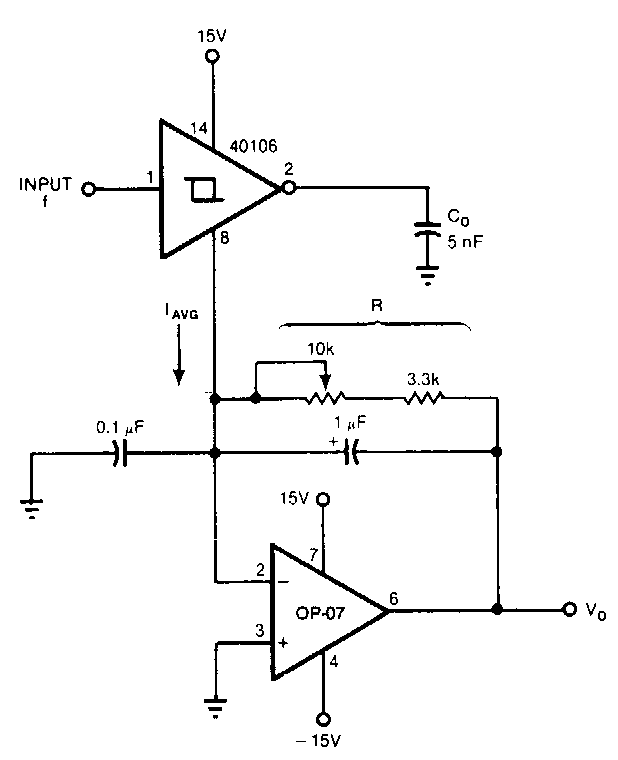The input frequency given to this converter can be in the range of 0-10 kHz. And the output can be between 0 to -10 V.

### Block Diagram of F-V converter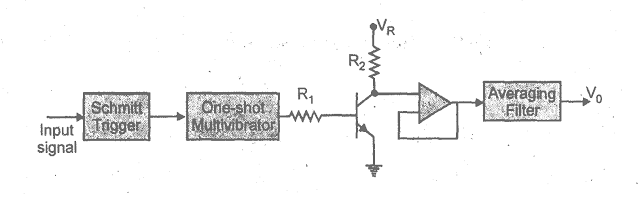The above block diagram shows a frequency to voltage converter. The circuit charges the capacitor to a certain level. An integrator is connected in it and the capacitor discharges into this integrator or a low pass circuit. This happens for all the cycles of the input waveform. The precision switch and the monostable multivibrator generate a pulse of a specific amplitude and period which is fed into the averaging network. Hence we get a DC voltage at the output.

### F-V CIRCUIT DIAGRAM USING LM331

This is the circuit diagram of frequency to voltage converter using LM331.

This IC is basically a voltage to frequency converter but it can be used as a frequency to voltage converter. Its applications also include A to D conversion and long term integration.

### FV converter WORKING

In this circuit, lm331 is used to convert frequency into voltage. The voltage on the output is proportional to the frequency at the input. It is an 8 pins IC. The source is connected to pin 8 and supplies 15V DC. Pins 3 and 4 are connected to ground. The input frequency is given at pin 6 and the output voltage is taken from pin 1. The input frequency is differentiated by using the resistor R7 and capacitor C3 and then the resultant pulse train goes to pin 6. The timer circuit gets triggered by the built-in comparator circuit in the IC when the negative edge of the pulse train appears at pin 6.

The current flowing out of pin 6 is proportional to the values of capacitor C1 and resistor R1 (which are also known as the timing components) and the input frequency. Therefore we get the output voltage across the resistor R4 which is proportional to the frequency of the input. 15V DC is used in this circuit but the operating voltage of IC can be between 5 volts to 30 volts DC. The value of the resistor R3 is dependent upon the supply voltage.

### APPLICATIONS OF F-V CONVERTERS

These converters are used in wide range of applications such as communication, power control, measurement and instrumentation systems etc.

We will discuss the following applications in detail:

1. Frequency to voltage converter in tachometers.
2. Frequency difference measurement.

#### F/V CONVERTER AND DIGITAL TACHOMETER

A digital tachometer is an electronic device which measures the rate of rotation of a wheel. They display the rate of rotation in the form of voltage which is why a frequency to voltage converter is required in them. The diagram below shows a digital tachometer.

#### A digital tachometer

The rate of occurrence of some events can be measured by a rate meter. It counts the events for a certain time period and then divides the number of events by the total time and hence we get a rate. This is the theory of operation of a simple tachometer.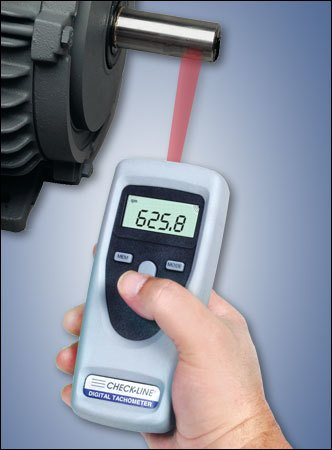We are using an IC LM2907 for this tachometer circuit. It is an 8 pins IC. At pin 1 we apply a frequency signal at the charge pump’s input. At pin 2 the voltage will be between two values that are ¼ (VCC) – VBE and ¾ (VCC) – VBE.

The diagram below shows the configuration of the IC LM2907: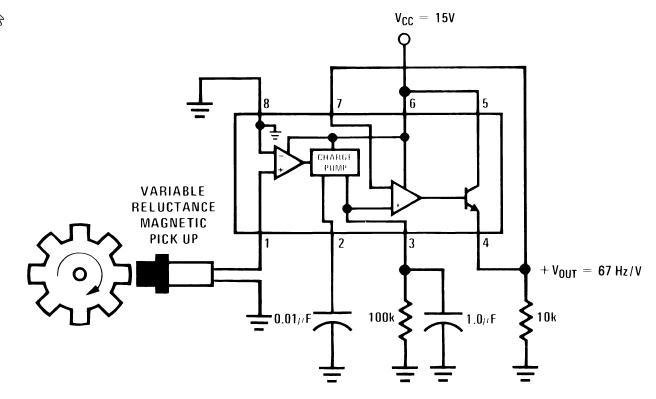The capacitors C1 and C2 and the resistor R1 have specific values according to the circuit requirements. These values can be studied from the data sheet of LM2907.

#### Interfacing of LM2907

The input signal is given at pin 1 and at pin 11 we apply a reference voltage. Pin 8 and pin 9 is supplied with a constant voltage. The op amp’s inverting input is connected with the output of the emitter. We get a low impedance voltage at the pin 5 which is proportional to the given input frequency. From the pin 5 and pin 10 we get the output signal of 67 Hz/V. This output is sent to the ADC and then the DSP can read this output.

#### FREQUENCY DIFFERENCE MEASUREMENT

TC9400 is a frequency to voltage and voltage to frequency converter IC. Its basic circuit connections include three resistors, two capacitors and reference voltage. We can use two TC9400 ICs and operate both of them in the mode of frequency to voltage conversion in order to obtain the frequency difference measurements.

We use two converters and we get V1 and V2 as two separate outputs. A unity gain inverts the voltage V2 coming from the 2nd F/V converter.  An op amp is connected which adds both the voltages V1 and the inverted –V2 voltage. This sum will be proportional to the actual frequency difference between F1 and F2. A V/F converter is also connected in the circuit which gives the frequency output which is again proportional to the frequency difference between F1 and F2. Hence we get the frequency difference measurement in terms of frequency as well as in terms of voltages.  The diagram below shows the circuit of frequency difference measurement.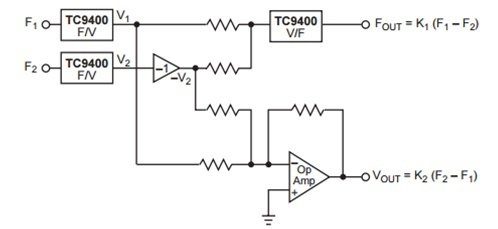Besides these two applications, there are numerous other applications of F/V converters such as frequency divider/multipliers, frequency decoders, frequency meters and motor speed control etc which can easily be found on several web pages.

### 10 thoughts on “FREQUENCY TO VOLTAGE CONVERTER CIRCUITS”

1. I enjoyed reading the content of it. I was amazed by it.
Keep it up. Love to see more post from you. Thank you for sharing.

• yes thanks

2. I have one question….ic 40106 ground pin that is 7 is not mentioned in the figure plz help me out im preparing the same circut….

3. The question above by Kamra refrs to the first schematic.
I think that pin 7 of the IC 40106 is used and thet “8” is mistyped.

4. Hi Mr. Bilal,
I interested in F/V converter, is it possible that for me to change the value of external resistors and capacitors in the figure (F-V CIRCUIT DIAGRAM USING LM331) so that the Vout is in between 0-5v with fin (input frequency) in between 0-100hz?

5. Hi Mr. Bilal,
I interested also in F/V converter, is it possible that for me to change the value of external resistors and capacitors in the figure (F-V CIRCUIT DIAGRAM USING LM331) so that the Vout is in between 0-5v with fin (input frequency) in between 0-100hz? please email me to [email protected]
john

6. Can it be arranged for an input frequency of 30KHz and an out put voltage of 30 or more VDc ?

7. Hello Mr Bilal,
same question asked by John: I’d like to modify the LM331 circuit for input frequency range 0-30 Hz and output voltage 0 to 5V = VDC power supply.
I would need 0 V at 0 Hz (I evaluated also circuits with Sample & Hold, but Vout would be unpredictable when not sampling)
Thanks if you can help me, please write in case to [email protected]
Thanks a lot
Paolo